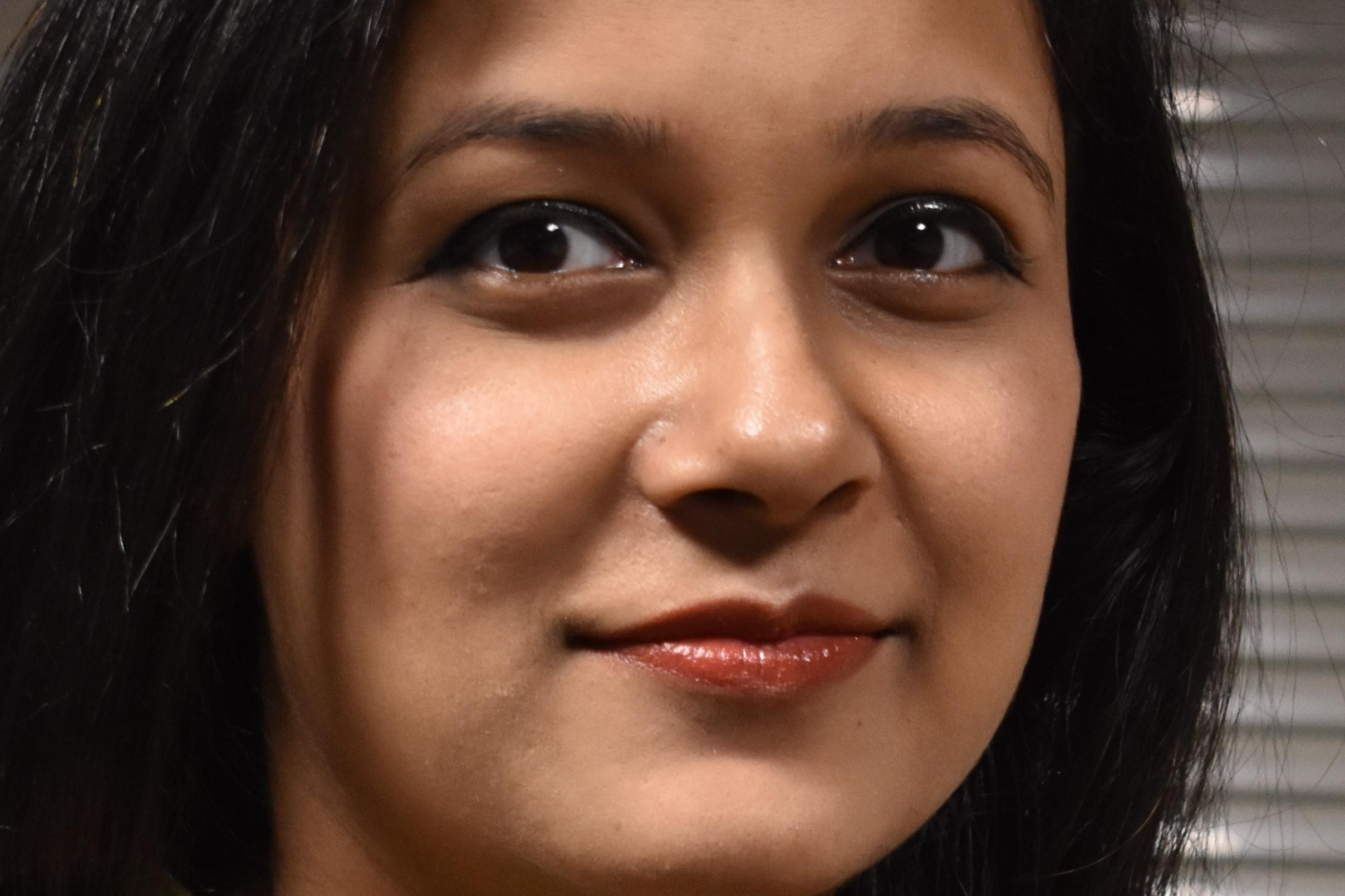Post Date
Sep 19 2021

## Development of Novel Techniques for Signal Acquisition and Representation on Spherical Manifolds

Year
2020
Supervisor:
Dr. Zubair Khalid
Students:
Wajeeha Nafees
Reference / Filters
Electrical Engineering
Abstract:
The study of spherical signal processing methods enables signal analysis in a variety of diverse fields of science and engineering including, but not limited to, planetary sciences, geophysics, acoustics, medical imaging, quantum mechanics, etc. The proposed dissertation is thus directed towards processing and analysis of signals on spherical objects: the sphere and the ball.

The first part of the thesis focuses on the study of the Slepian concentration problem on the sphere and the ball. We present a generalized formulation of the Slepian concentration problem on the sphere for finding band-limited functions with an optimal concentration in the spatial domain. By introducing weighting functions in the formulation of classical Slepian concentration problem and assigning different values to these weighting functions, we present two variants of the concentration problem namely the differential and the weighted Slepian concentration problem. For the Slepian problem on the ball, we design a new set of optimal basis functions with an optimality criterion that the bases are simultaneously concentrated in both the spatial and spectral domains. The optimal basis functions are designed as a linear combination of space-limited functions with maximal concentration in the spectral region and band-limited functions with maximal concentration in the spatial region.

In the second part of this thesis, we focus our attention on sampling schemes on the sphere. It is desirable for a sampling scheme and its associated spherical harmonic transform (SHT) algorithm to utilize the least number of samples, exhibit stability, be computationally efficient and have low complexity in order to exactly or accurately represent a band-limited signal on the sphere. We develop novel methods to improve one or more of the aforementioned attributes of the existing sampling schemes on the sphere. For the optimal-dimensionality sampling scheme, we propose the placement of samples on the sphere such that the matrices involved in the computation of the SHT are well-conditioned, and develop an iterative algorithm which reduces the error by a factor of 10~100. Using the proposed method, we also investigate the error in such case when only a small part of the sphere is inaccessible to support the signal analysis in applications (e.g., geophysics, cosmology, acoustics) where samples cannot be taken over the whole sphere due to practical limitations. We further propose an antipodally symmetric sampling scheme of optimal dimensionality for the sampling of band-limited signals. The proposed scheme takes (asymptotically) L2 number of samples for the sampling of spherical signal of band-limit L and computing its SHT accurately. Since the number of samples are asymptotically equal to the degrees of freedom of the signal in harmonic space, the proposed scheme attains optimal spatial dimensionality. We also formulate the SHT associated with proposed sampling scheme. We employ the antipodal symmetry of the sampling points which is exploited to separate the signal into antipodally symmetric and asymmetric components due to which the signal splits in harmonic space into the signals of even and odd spherical harmonic degrees. The exploitation of this splitting in the formulation of the SHT makes our method computationally efficient by a factor of four in comparison with the existing methods developed for sampling schemes that attain optimal spatial dimensionality. Lastly, to support the applications where the measurements can only be taken over spatially limited region on the sphere due to practical limitations, we design a spatially-limited sampling scheme on the sphere for the computation of SHT of band-limited signals. By enclosing the inaccessible region with an (anisotropic) ellipsoidal region followed by the rotation of the region to the pole or the equator, we propose an iso-latitude sampling scheme on the sphere. We also present a method to place the samples over the spatially-limited region such that the SHT can be computed accurately.

We also present a variant of the equiangular sampling scheme which requires fewer number of samples as compared to the current schemes: an exact method with a slight increase in complexity of the associated SHT algorithm.